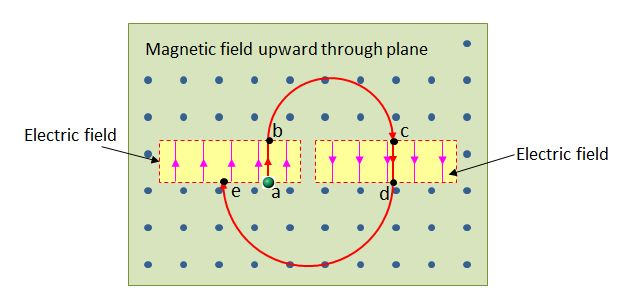# Moving Charged ParticleThe above diagram shows a charged particle starting from position a, moving through two separate uniform electric fields in a uniform magnetic field, and eventually arriving at e. The direction of the magnetic field is upward, vertical to the plane. The path that the charged particle moves along is a $\to$ b $\to$ c $\to$ d $\to$ e. Which of the following explanations is correct?

a) The charged particle is positively charged.
b) The speed of the charged particle from position b to position c is constant.
c) The speed of the charged particle at position d is larger than that at position b.

×

Problem Loading...

Note Loading...

Set Loading...